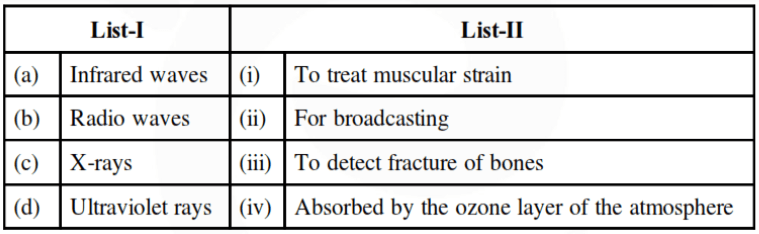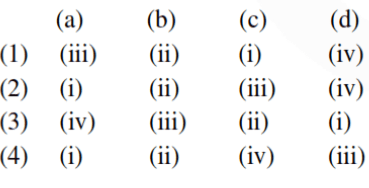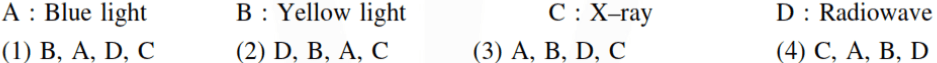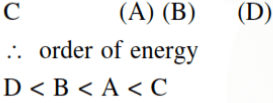Most Affordable JEE | NEET | 8,9,10 Preparation by Kota's Top IITian Doctor Faculties

# Electromagnetic Wave - JEE Main Previous Year Questions with Solutions`
JEE Main Previous Year Question of Physics with Solutions are available here. Practicing JEE Main Previous Year Papers Questions of Physics will help all the JEE aspirants in realizing the question pattern as well as help in analyzing their weak & strong areas. Get detailed Class 11th &12th Physics Notes to prepare for Boards as well as competitive exams like IIT JEE, NEET etc. eSaral helps the students in clearing and understanding each topic in a better way. eSaral is providing complete chapter-wise notes of Class 11th and 12th both for all subjects. Besides this, eSaral also offers NCERT Solutions, Previous year questions for JEE Main and Advance, Practice questions, Test Series for JEE Main, JEE Advanced and NEET, Important questions of Physics, Chemistry, Math, and Biology and many more. Download eSaral app for free study material and video tutorials. Simulator Previous Years AIEEE/JEE Mains Questions
Q. If a source of power $4 \mathrm{kW}$ produces $10^{20}$ photons/second, the radiation belongs to a part of the spectrum called (1) microwaves (2) y-rays (3) X-rays (4) ultraviolet rays [AIEEEE 2010]
Ans. (3) Power $=\frac{\text { nhc }}{\lambda} \lambda \approx 1 \mathrm{A} \equiv \mathrm{X}$ Rays
Q. An electromagnetic wave in vacuum has the electric and magnetic fields $\overrightarrow{\mathrm{E}}$ and $\overrightarrow{\mathrm{B}}$, which are always perpendicular to each other. The direction of polarization is given by $\overrightarrow{\mathrm{X}}$ and that ofwave propagation by $\overrightarrow{\mathrm{k}}$. Then (1) $\overrightarrow{\mathrm{X}} \| \overrightarrow{\mathrm{B}}$ and $\overrightarrow{\mathrm{k}} \| \overrightarrow{\mathrm{E}} \times \overrightarrow{\mathrm{B}}$ (2) $\overrightarrow{\mathrm{X}} \| \overrightarrow{\mathrm{E}}$ and $\overrightarrow{\mathrm{k}} \| \overrightarrow{\mathrm{B}} \times \overrightarrow{\mathrm{E}}$ (3) $\overrightarrow{\mathrm{X}} \| \overrightarrow{\mathrm{B}}$ and $\overrightarrow{\mathrm{k}} \| \overrightarrow{\mathrm{B}} \times \overrightarrow{\mathrm{E}}$ (4) $\overrightarrow{\mathrm{X}} \| \overrightarrow{\mathrm{E}}$ and $\overrightarrow{\mathrm{k}} \| \overrightarrow{\mathrm{E}} \times \overrightarrow{\mathrm{B}}$ AIEEE-2012
Ans. (4)
Q. Match List-I (Electromagnetic wave type) with List-II (Its association/application) and select the correct option from the choices given below the lists:[JEE- Mains 2014]
Ans. (2)
Q. During the propagation of electromagnetic waves in a medium: (1) Electric energy density is equal to the magnetic energy density (2) Both electric magnetic energy densities are zero (3) Electric energy density is double of the magnetic energy density (4) Electric energy density is half of the magnetic energy density. [JEE- Mains 2014]
Ans. (1)
Q. A red LED emits light at 0.1 watt uniformly around it. The amplitude of the electric field of the light at a distance of 1 $\mathrm{m}$ from the diode is :- (1) $5.48 \mathrm{V} / \mathrm{m}$ (2) $7.75 \mathrm{V} / \mathrm{m}$ (3) $1.73 \mathrm{V} / \mathrm{m}$ (4) $2.45 \mathrm{V} / \mathrm{m}$ [JEE- Mains 2015]
Ans. (4) $\mathrm{I}_{\mathrm{av}}=\frac{1}{2} \varepsilon_{0} \mathrm{E}^{2} \mathrm{C}=\frac{\mathrm{P}}{4 \pi \mathrm{r}^{2}}$ $\mathrm{E}=\sqrt{\frac{2 \mathrm{P}}{4 \pi \mathrm{r}^{2} \varepsilon_{0} \mathrm{c}}}$ On putting value we get $=2.45 \mathrm{v} / \mathrm{m} .$
Q. Arrange the following electromagnetic radiations per quantum in the order of increasing energy :-[JEE- Mains 2016]
Ans. (2) Energy $=\frac{\mathrm{hc}}{\lambda}$ order of wavelength $x$ ray, VIBGYOR, RadiowavesManik agarwal
Feb. 6, 2021, 12:24 a.m.
Isme to latest question nhi h sir ......
ABCD
Dec. 22, 2020, 7:57 p.m.
Not enough questions in this auch a stupid and worst app ....
Oct. 23, 2020, 8:38 p.m.
More concept solutions required
Ninte appan
Oct. 18, 2020, 4:23 p.m.
More problems required
Sudish
Oct. 9, 2020, 7:46 p.m.
Not so useful and better add more problems
Ullu Ka Pattha
Aug. 31, 2020, 7:11 p.m.
Bsdk aur questions Dal randi
Cerry Mara Maa Ha
Aug. 29, 2020, 3:16 p.m.
Madarchod website. Chutiye developer, baki saare questions kaha gaye?
Name
Aug. 11, 2020, 11:45 a.m.